Comparing Analog and Digital Complex Planes Next  |  Prev  |  Up  |  Top  |  Index  |  JOS Index  |  JOS Pubs  |  JOS Home  |  Search

### Comparing Analog and Digital Complex Planes

In signal processing, it is customary to use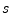as the Laplace transform variable for continuous-time analysis, and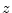as the-transform variable for discrete-time analysis. In other words, for continuous-time systems, the frequency domain is theplane'', while for discrete-time systems, the frequency domain is theplane.'' However, both are simply complex planes.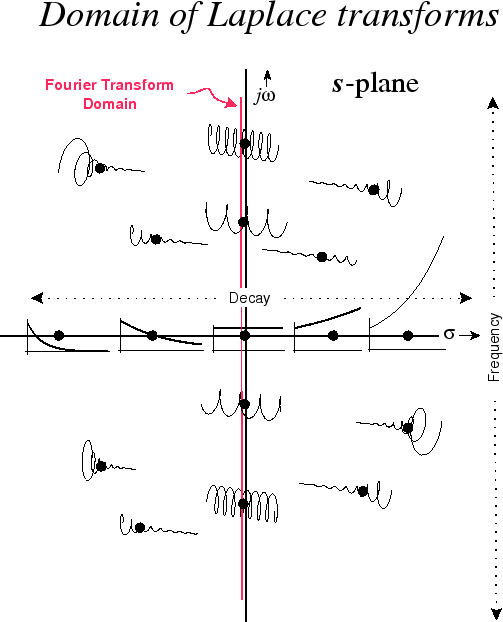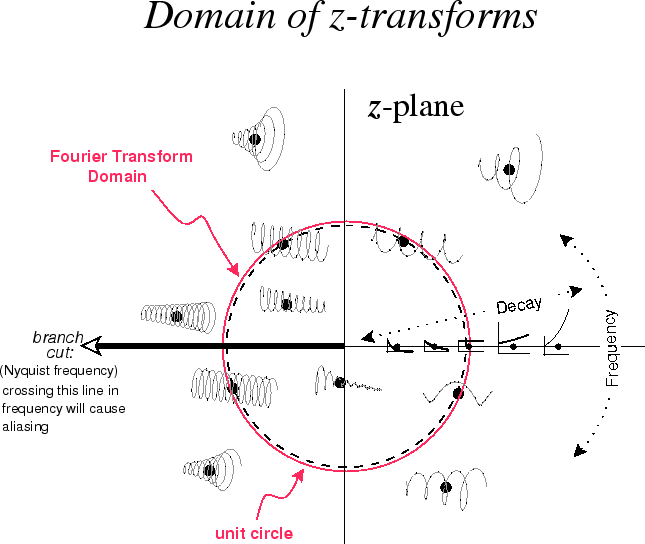Figure 4.18 illustrates the various sinusoids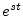represented by points in theplane. The frequency axis is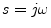, called the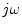axis,'' and points along it correspond to complex sinusoids, with dc at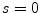(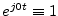). The upper-half plane corresponds to positive frequencies (counterclockwise circular or corkscrew motion) while the lower-half plane corresponds to negative frequencies (clockwise motion). In the left-half plane we have decaying (stable) exponential envelopes, while in the right-half plane we have growing (unstable) exponential envelopes. Along the real axis (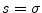), we have pure exponentials. Every point in theplane corresponds to a generalized complex sinusoid,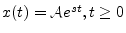, with special cases including complex sinusoids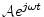, real exponentials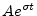, and the constant function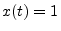(dc).

Figure 4.19 shows examples of various sinusoids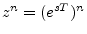represented by points in theplane. The frequency axis is the unit circle''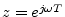, and points along it correspond to sampled complex sinusoids, with dc at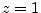(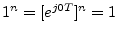). As in theplane, the upper-half plane corresponds to positive frequencies while the lower-half plane corresponds to negative frequencies. Inside the unit circle, we have decaying (stable) exponential envelopes, while outside the unit circle, we have growing (unstable) exponential envelopes. Along the positive real axis ( re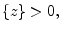im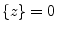), we have pure exponentials, but along the negative real axis ( re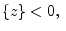im), we have exponentially enveloped sampled sinusoids at frequency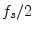(exponentially enveloped alternating sequences). The negative real axis in theplane is normally a place where all signaltransforms should be zero, and all system responses should be highly attenuated, since there should never be any energy at exactly half the sampling rate (where amplitude and phase are ambiguously linked). Every point in theplane can be said to correspond to sampled generalized complex sinusoids of the form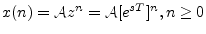, with special cases being sampled complex sinusoids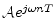, sampled real exponentials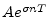, and the constant sequence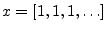(dc).

In summary, the exponentially enveloped (generalized'') complex sinusoid is the fundamental signal upon which other signals are projected'' in order to compute a Laplace transform in the continuous-time case, or atransform in the discrete-time case. As a special case, if the exponential envelope is eliminated (set to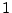), leaving only a complex sinusoid, then the projection reduces to the Fourier transform in the continuous-time case, and either the DFT (finite length) or DTFT (infinite length) in the discrete-time case. Finally, there are still other variations, such as short-time Fourier transforms (STFT) and wavelet transforms, which utilize further modifications such as projecting onto windowed complex sinusoids.

Next  |  Prev  |  Up  |  Top  |  Index  |  JOS Index  |  JOS Pubs  |  JOS Home  |  Search

[How to cite this work] [Order a printed hardcopy]